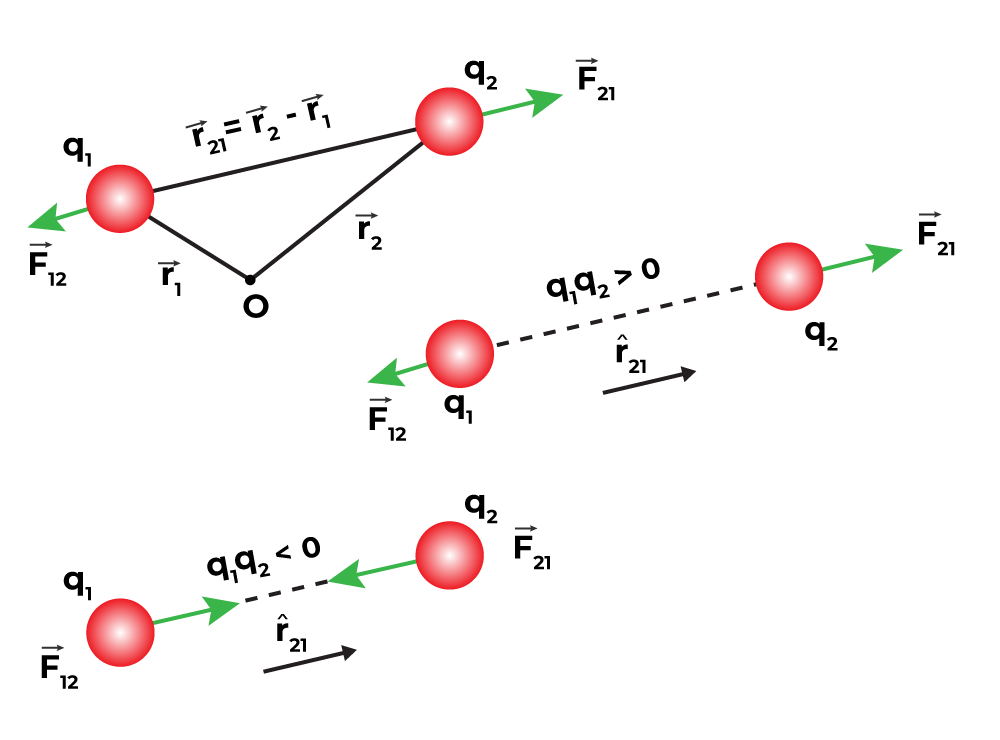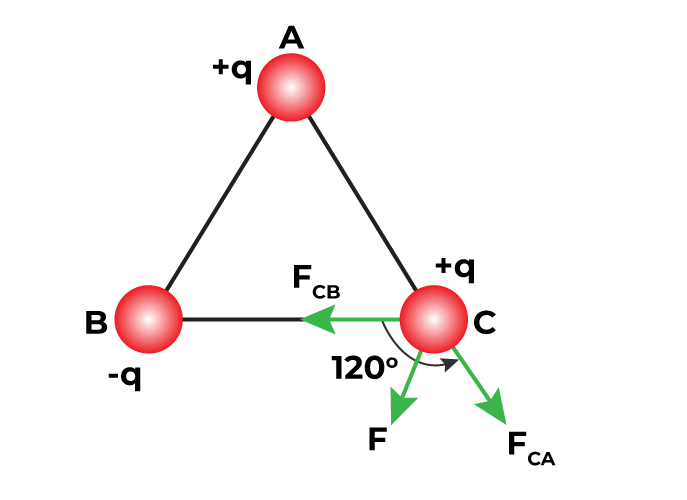GeeksforGeeks App
Open AppBrowser
Continue

## Related Articles

Coulomb’s law is defined as a mathematical concept that defines the electric force between charged objects. Columb’s Law states that the force between any two charged particles is directly proportional to the product of the charge but is inversely proportional to the square of the distance between them. Let’s learn about Columb’s law in detail in this article.

## What is Coulomb’s Law?

Coulomb’s law is a mathematical formula that describes the force between two point charges. When the size of charged bodies is substantially smaller than the separation between them, then the size is not considered or can be ignored. The charged bodies can be considered point charges.

The force of attraction or repulsion between two charged things is directly proportional to the product of their charges and inversely proportional to the square of the distance between them, according to Coulomb’s law. It acts along the line that connects the two charges that are regarded to be point charges.

Coulomb studied the force between two point charges and found that it is inversely proportional to the square of the distance between them, directly proportional to the product of their magnitudes, and acting in a line that connects them.

## History of Coulomb’s Law

Charles Augustin de Coulomb a french mathematician in 1785 first describes a force between two charged bodies in mathematical equations. He stated that the charge bodies repel or attract each other accordingly based on their charge, i.e. opposite charge attracts each other and similar charge repels. He also states the mathematical formula for the force between them, which is called Columb’s Law.

## Coulomb’s Law Formula(Scalar Form)

As we know, the force (F) between two point charges q1 and q2 separated by a distance r in a vacuum is,

Proportional to the product of the charges.

F ∝ q1q2

Inversely Proportional to the square of the distance between them,

F ∝ 1/r2

F ∝ q1q2 / r2

then,

F = k q1q2 / r2

where,
k is proportionality constant and equals to 1/4πε0.
Symbol ε0 is permittivity of a vacuum.
Value of k is 9 × 109 Nm2/ C2 {when we take the S.I unit of value of ε0 is 8.854 × 10-12 C2 N-1 m-2.}

## Coulomb’s Law in Vector Form

Coulomb’s law is better written in vector notation because force is a vector quantity. Charges q1 and q2 have location vectors r1 and r2, respectively. F12 denotes force on q1 owing to q2 and F21 denotes force on q2 owing to q1. For convenience, the two-point charges q1 and q2 have been numbered 1 and 2, respectively, and the vector leading from 1 to 2 has been designated by r21.Similarly, the vector leading from 2 to 1 is denoted by r12,r21 and r12 are the magnitudes of the vectorsand, respectively and magnitude r12 is equal to r21. A unit vector along the vector specifies the vector’s direction. The unit vectors are used to denote the direction from 1 to 2 (or 2 to 1). The unit vectors define as,Similarly,Coulomb’s force law between two point charges q1 and q2 located at vector r1 and r2 is then expressed as,## Key Points on Coulomb’s Law

• Coulomb’s Law holds true regardless of whether q1 and q2 are positive or negative. F21 is toward, which is a repulsive force, as it should be for like charges that are if q1 and q2 are of the same sign (either both positive or both negative). When the signs of q1 and q2 are opposite or dislike charges, F21 is toward, that is towardwhich shows attraction, as expected for dissimilar charges. As a result, we don’t need to construct separate equations for like and unlike charges. Both instances are handled correctly by the above expression for Coulomb’s force law.
• Coulomb’s force law can be used to calculate the force F12 on charge q1 due to charge q2 by simply swapping 1 and 2 as,• Coulomb’s law agrees with Newton’s third law.
• In a vacuum, Coulomb’s law expression determines the force between two charges q1 and q2. If the charges are deposited in matter or there is matter in the intervening area, the situation becomes more complicated due to the presence of charged matter constituents.
• Two identical conductors with charges q1 and q2 are brought into contact and subsequently separated, resulting in each conductor having a charge equal to (q1+q2)/2. Each charge will be equal to (q1-q2)/2 if the charges are q1 and –q2.

## What is 1 Coulomb of Charge?

Columb is the SI unit of charge. If a charge repels an equal charge of the same sign with a force of 9×109 N the charge is of 1 Coulomb given so the charges are one meter apart in a vacuum.

1 coulomb is a bigger unit of charge and is not used in daily life. We use smaller units such as micro coulomb, etc.

## Conditions for Stability of Coulomb’s Law

If two charges are arranged in a straight line AB and one charge q is slightly displaced towards A, the force acting on A FA increases in magnitude while the force acting on B FB decreases in magnitude. Thus, the net force on q shifts towards A.  So we can say that for axial displacement, the equilibrium is unstable.

If q is displaced perpendicular to line AB, the force FA and FB are changed in such a manner that they bring the charge to its original position. Now we can say that for perpendicular displacement, the equilibrium is stable.

## Applications of Coulomb’s Law

Coulomb’s Law is one of the basic laws of Physics. It is used for various purposes, some of its important applications are discussed below,

• It is used to calculate the distance and force between the two charges.
• It is used to arrange the charges in stable equilibrium.
• Columbus law is used to calculate electric field.

Electric field is given by,

E = F / QT (N/C)

where,
E is the Strength of the electric field
F is the Electrostatic force
QT is the Test charge measured in coulombs

## Limitations of Coulomb’s Law

There are some limitations of Coulomb’s Law which are discussed below in the article,

• Coulomb’s Law is applicable for the point charges which are at rest.
• Coulomb’s Law is only applicable in situations where the inverse square law is followed.
• Coulomb’s Law is applicable only for the charges which are considered to be spherical. For charges with arbitrary shapes, Coulomb’s Law is not applicable because we cannot determine the distance between the charges.

Also, Check

## Solved Numerical Example on Coulomb’s Law

Example 1: Charges of magnitude 100 micro coulombs each are located in a vacuum at the corners A, B and C of an equilateral triangle measuring 4 meters on each side. If the charge at A and C are positive and the charge at B negative, what is the magnitude and direction of the total force on the charge at C?

Solution:The Force FCA is applied toward AC and the expression for the FCA is expressed asSubstitute the values in the above expression,The Force FCB is applied toward CB and the expression for the FCB is expressed asSubstitute the values in the above expression,Therefore, the two forces are equal in magnitude but in different directions. The angle between them is 120º. The resultant force F is given by,Example 2: A positive charge of 6×10-6 C is 0.040 m from the second positive charge of 4×10-6 C. Calculate the force between the charges.

Solution:

Given,

First charge q1 = 6×10-6 C.

Second charge q2 = 4×10-6 C.

Distance between the charges r = 0.040 m

k = 9×109

We know that, F = k q1q2 / r2

Substitute the values in the above expression,

F = k q1q2 / r2

F = 9×109×[(6×10-6)× (4×10-6)] / (0.04)2

F= 134.85 N

Example 3: Two-point charges, q1 = +9 μC and q2 = 4 μC are separated by a distance r = 12 cm. What is the magnitude of the electric force?

Solution:

Given

k = 8.988 x 109 Nm2C−2

q1 = 5 ×10-6 C

q2 = 6 ×10-6 C

r = 14 cm = 0.14 m

Fe = k × (q1q2) / r2

Fe = [8.988 × 109 × (5 ×10-6 C) × (6 ×10-6 C)] / (0.14)2

Fe = 269.64 / (0.0196)

Fe = 13.75 N

For more Problems on Coulomb’s Law

## FAQs on Coulomb’s Law

### Question 1: Define Coulomb’s Law.

The electrostatic force of attraction or repulsion between two stationary point charges is directly proportional to the product of the magnitudes of the charges and inversely proportional to the square of the distance between them i.e.

F ∝ q1q2 / r2

### Question 2: State Coulomb’s Inverse-square law in electrostatics.

Coulomb’s Inverse-square law in electrostatics states that the force applied between two electrically charged particles is inversely proportional to the square of the distance between two particles.

### Question 3: What is one coulomb of charge?

If one ampere of current passes through a conductor in one second then the charge transfer is one coulomb. One coulomb charge is also defined as the charge carried by 6×1018 electrons.

### Question 4: Is electrostatic force between any two point charges a central force?

Yes. The electrostatic force between two point charges always acts along the line joining the two charges. Hence it is a central force.

### Question 5: What are the Conditions for the Stability of Coulomb’s Law?

The condition for stability of Coulomb’s Law is if a charge is introduced in a stable system of n charges then it is placed in such a position that the force on the test charge through all the other charges must cancel out each other.